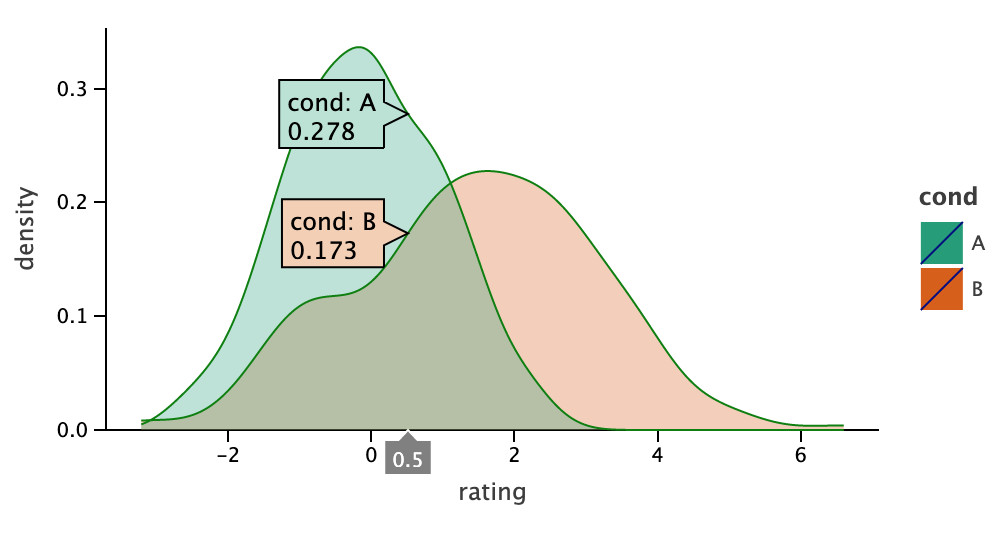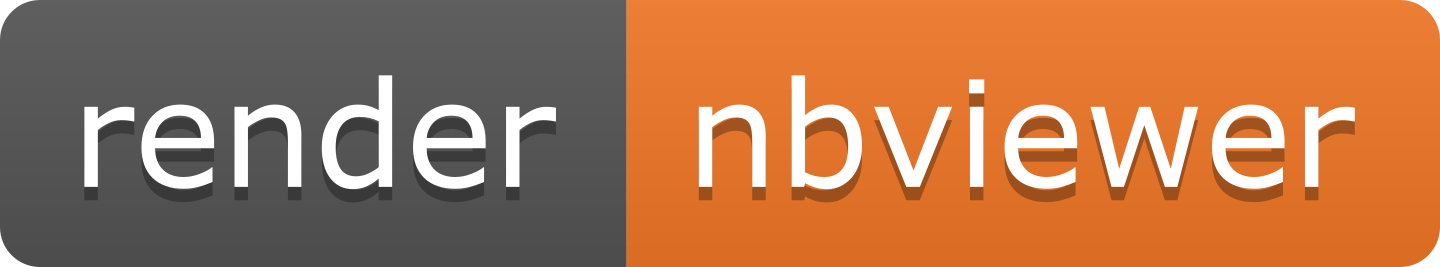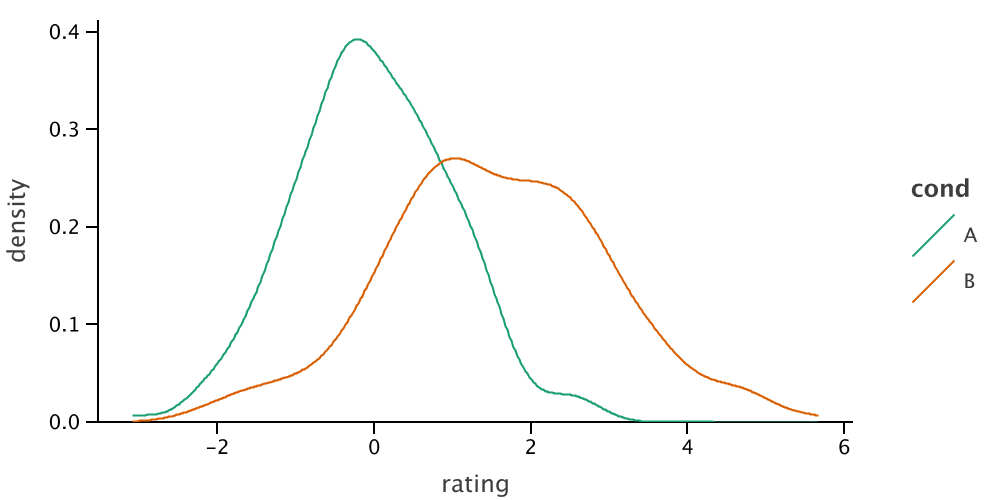Need help with lets-plot-kotlin?
Click the “chat” button below for chat support from the developer who created it, or find similar developers for support.JetBrains221 Stars22 ForksOther500 Commits10 Opened issues#### Description

Kotlin API for Lets-Plot - an open-source plotting library for statistical data.!
?

#### Need anything else?# Lets-Plot for KotlinLatest Lets-Plot Kotlin API VersionLatest Lets-Plot VersionLicense## Overview

Lets-Plot for Kotlin is a Kotlin API for the Lets-Plot library - an open-source plotting library for statistical data.

Lets-Plot Kotlin API is built on the principles of layered graphics first described in the Leland Wilkinson work The Grammar of Graphics and later implemented in the ggplot2 package for R.

This grammar [...] is made up of a set of independent components that can be composed in many different ways. This makes [it] very powerful because you are not limited to a set of pre-specified graphics, but you can create new graphics that are precisely tailored for your problem. - Hadley Wickham, "ggplot2: Elegant Graphics for Data Analysis"

Read Lets-Plot Usage Guide for quick introduction to the Grammar of Graphics and Lets-Plot Kotlin API.

## Lets-Plot in Jupyter with Kotlin Kernel

#### Installation

In Jupyter notebook with a Kotlin Kernel, Lets-Plot library is available out-of-the-box. To install Kotlin Kernel and OpenJDK into a Conda environment, run the following command:

```shell script
conda install kotlin-jupyter-kernel -c jetbrains
```

#### "Line Magics"

You can include all the necessary Lets-Plot boilerplate code to a notebook using the following "line magic":

```%use lets-plot
```

This will apply the lets-plot
`library descriptor`
bundled with the Kotlin Jupyter Kernel installed in your environment.

The

`%useLatestDescriptors`
line magic will force Kotlin Kernel to pull and apply the latest repository version of all
`library descriptors`
.

You can override lets-plot

`library descriptor`
settings using the lets-plot line magic parameters, like:
```%use lets-plot(api=1.1.0, lib=1.5.4, js=1.5.4, isolatedFrame=false)
```

Where: -
`api`
- version of Lets-Plot Kotlin API. -
`lib`
- version of Lets-Plot library (JAR-s). -
`js`
- version of Lets-PLot JavaScript bundle. -
`isolatedFrame`
- If
`false`
: load JS just once per notebook (default in Jupyter). If
`true`
: include Lets-Plot JS in each output (default in Datalore notebooks)

See: Line Magics documentation in the Kotlin Jupyter project for more details.

#### Quickstart in Jupyter

• In Jupyter, create a new notebook and choose the Kotlin kernel (see the instructions for more details on how to select a kernel).

• Add the following code to a Jupyter notebook:

```%use lets-plot
```
```val rand = java.util.Random()
val data = mapOf(
"rating" to List(200) { rand.nextGaussian() } + List(200) { rand.nextGaussian() * 1.5 + 1.5 },
"cond" to List(200) { "A" } + List(200) { "B" }
)

var p = lets_plot(data)
p += geom_density(color="dark_green", alpha=.3) {x="rating"; fill="cond"}
p + ggsize(500, 250)
```

• Execute the added code to evaluate the plotting capabilities of Lets-Plot.#### Example of notebooks

Try the following examples to study features of the

`Lets-Plot`
library.

## Lets-Plot-Kotlin in Datalore notebooks

Datalore is an online data science notebook by JetBrains.

In Datalore notebook you can run Kotlin code directly in your browser. Many popular Kotlin libraries are preinstalled and readily available (see the list of supported Kotlin libraries).

Watch the Datalore Getting Started Tutorial video for a quick introduction to Datalore.

## Lets-Plot in JVM and Kotlin/JS application

Apart from Jupyter notebooks, Lets-Plot library and Kotlin API enables embedding plots into a JVM and a Kotlin/JS application.

In the lets-plot-mini-apps GitHub repository you will find examples of using Lets-Plot Kotlin API in JVM and Kotlin/JS projects.

## Lets-Plot Kotlin API

#### Data sampling

Sampling is a special technique of data transformation, which helps to deal with large datasets and overplotting.

#### GGBunch

GGBunch allows to show a collection of plots on one figure. Each plot in the collection can have arbitrary location and size. There is no automatic layout inside the bunch.

Examples:

#### Saving plot to a file

The

`ggsave()`
function is a convenient way of saving a plot or a GGBunch object to a file.

The supported export formats are:

`SVG, HTML, PNG, JPEG and TIFF`
.

For example, the code below will save plot as a PNG image to the file

`//lets-plot-images/density.png`
:
```%use lets-plot

val rand = java.util.Random(123)
val n = 400
val data = mapOf (
"rating" to List(n/2) { rand.nextGaussian() } + List(n/2) { rand.nextGaussian() * 1.5 + 1.5 },
"cond" to List(n/2) { "A" } + List(n/2) { "B" }
)
var p = lets_plot(data) +
geom_density { x = "rating"; color = "cond" } + ggsize(500, 250)
ggsave(p, "density.png")```See

`ggsave()`

#### Tooltip customization

You can customize the content of tooltips for the layer by using the parameter

`tooltips`
of
`geom`
functions.

#### GeoTools support

GeoTools is an open source Java GIS Toolkit.

Lets-Plot supports visualization of a set of

`SimpleFeature`
-s stored in
`SimpleFeatureCollection`
, as well as individual
`Geometry`
and
`ReferencedEnvelope`
objects.

## What is new in 3.0.2

• Ordering categories:

`asDiscrete()`
function: -
`orderBy`
- name of the variable by which the ordering will be performed; -
`order`
- ordering direction: 1 for ascending direction and -1 for descending (default).

See: as_discrete.md.

• Other improvements and fixes - see CHANGELOG.md for details.

## Change Log

See CHANGELOG.md.